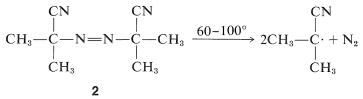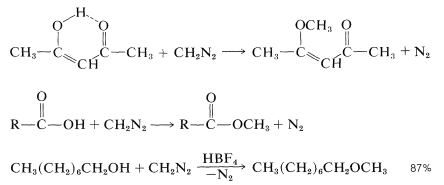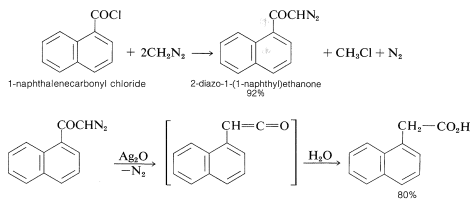# 24.7: Some Compounds with N-N Bonds

$$\newcommand{\vecs}{\overset { \rightharpoonup} {\mathbf{#1}} }$$ $$\newcommand{\vecd}{\overset{-\!-\!\rightharpoonup}{\vphantom{a}\smash {#1}}}$$$$\newcommand{\id}{\mathrm{id}}$$ $$\newcommand{\Span}{\mathrm{span}}$$ $$\newcommand{\kernel}{\mathrm{null}\,}$$ $$\newcommand{\range}{\mathrm{range}\,}$$ $$\newcommand{\RealPart}{\mathrm{Re}}$$ $$\newcommand{\ImaginaryPart}{\mathrm{Im}}$$ $$\newcommand{\Argument}{\mathrm{Arg}}$$ $$\newcommand{\norm}{\| #1 \|}$$ $$\newcommand{\inner}{\langle #1, #2 \rangle}$$ $$\newcommand{\Span}{\mathrm{span}}$$ $$\newcommand{\id}{\mathrm{id}}$$ $$\newcommand{\Span}{\mathrm{span}}$$ $$\newcommand{\kernel}{\mathrm{null}\,}$$ $$\newcommand{\range}{\mathrm{range}\,}$$ $$\newcommand{\RealPart}{\mathrm{Re}}$$ $$\newcommand{\ImaginaryPart}{\mathrm{Im}}$$ $$\newcommand{\Argument}{\mathrm{Arg}}$$ $$\newcommand{\norm}{\| #1 \|}$$ $$\newcommand{\inner}{\langle #1, #2 \rangle}$$ $$\newcommand{\Span}{\mathrm{span}}$$$$\newcommand{\AA}{\unicode[.8,0]{x212B}}$$

Among the organic nitrogen compounds having nitrogen above the oxidation level of ammonia are a wide variety of substances with $$\ce{N-N}$$ bonds. We shall mention only a very few of the more important of these substances: hydrazines, azo and diazo compounds, and azides.

## Hydrazines

Organic hydrazines or diazanes are substitution products of $$\ce{NH_2-NH_2}$$ and have many properties similar to those of amines in being basic and forming acyl derivatives as well as undergoing alkylation and condensations with carbonyl compounds (Section 16-4C). Unsymmetrical hydrazines can be prepared by careful reduction of $$\ce{N}$$-nitrosamines. 1,1-Dimethyldiazane is prepared in this way for use as a rocket fuel:Aromatic hydrazines are best prepared by reduction of aromatic diazonium salts (Table 23-4).

Hydrazines of the type $$\ce{R-NH-NH-R}$$ are easily oxidized to the corresponding azo compounds, $$\ce{R-N=N-R}$$. With nitrous acid, monosubstituted hydrazines are converted to azides:

$\ce{R-NH-NH_2} + \ce{HONO} \rightarrow \ce{R-N=} \overset{\oplus}{\ce{N}} \ce{=} \overset{\ominus}{\ce{N}} + 2 \ce{H_2O}$

## Azo Compounds

Azo or diazo compounds possess the $$\ce{-N=N}-$$ grouping. Aliphatic azo compounds of the type $$\ce{R-N=N-H}$$ appear to be highly unstable and decompose to $$\ce{R-H}$$ and nitrogen. Derivatives of the type $$\ce{R-N=N-R}$$ are much more stable and can be prepared as mentioned above by oxidation of the corresponding hydrazines. Aromatic azo compounds are available in considerable profusion from diazo coupling reactions (Section 23-10C) and are of commercial importance as dyes and coloring materials.

A prime characteristic of azo compounds is their tendency to decompose into organic free radicals and liberate nitrogen:

$\ce{R-N=N-R} \rightarrow 2 \ce{R} \cdot + \ce{N_2}$

The ease of these reactions is usually a fairly reliable guide to the stabilities of the free radicals that result. For instance, it is found that dimethyldiazene (azomethane, $$\ce{CH_3N=NCH_3}$$) is stable to about $$400^\text{o}$$, and diphenyldiazene (azobenzene, $$\ce{C_6H_5N=NC_6H_5}$$) also is resistant to thermal decomposition; but, when the azo compound decomposes to radicals that have extra stability because of delocalization of the odd electron, the decomposition temperature is greatly reduced. Thus the azo compound, $$2$$, decomposes to radicals at moderate temperatures ($$60^\text{o}$$ to $$100^\text{o}$$), and for this reason is a very useful agent for generating radicals, such as those required for the initiation of polymerization of ethenyl compounds:## Diazo Compounds

The parent of the diazo compounds, diazomethane, $$\ce{CH_2=} \overset{\oplus}{\ce{N}} \ce{=} \overset{\ominus}{\ce{N}}$$, has been mentioned before in connection with ylide reactions for ring enlargement (Section 16-4A) and the preparation of methyl esters from acids (Table 18-7). It is one of the most versatile and useful reagents in organic chemistry, despite the fact that it is highly toxic, dangerously explosive, and cannot be stored without decomposition.

Diazomethane is an intensely yellow gas, bp $$-23^\text{o}$$, which customarily is prepared and used in diethyl ether or dichloromethane solution. It can be synthesized in a number of ways, the most useful of which employs the action of base on an $$\ce{N}$$-nitroso-$$\ce{N}$$-methylamide:As a methylating agent of reasonably acidic substances, diazomethane has nearly ideal properties. It can be used in organic solvents; reacts very rapidly without need for a catalyst (except with alcohols, which do require an acid catalyst); the coproduct is nitrogen which offers no separation problem; it gives essentially quantitative yields; and it act as as its own indicator to show when reaction is complete. With enols, it gives $$\ce{O}$$-alkylation:Besides being a methylating agent, diazomethane also is a source of $$\colon \ce{CH_3}$$ when irradiated with light. The carbene formed in this way is highly reactive and even will react with the electrons of a carbon-hydrogen bond to "insert" the carbon of the carbene between carbon and hydrogen. This transforms $$\ce{-C-H}$$ to $$\ce{-C-CH_3}$$:This $$\colon \ce{CH_3}$$ species is one of the most reactive reagents known in organic chemistry. Diazomethane undergoes a wealth of other unusual reactions. Besides those already mentioned are the following two examples:

Arndt-Eistert synthesis ($$\ce{COCl} \rightarrow \ce{-CH_2CO_2H}$$, Section 16-4A)Pyrazoline formation ([2 + 3] cycloaddition)The Arndt-Eistert synthesis is useful for converting an acid to the next higher member of the series. Pyrazolines are important intermediates for the preparation of cyclopropanes:Diazomethane originally was believed to possess the three-membered 1,2-diazacyclopropene ring structure, but this concept was disproved by electron-diffraction studies, which showed the linear structure to be correct:Recently, a variety of authentic 1,2-diazacyclopropenes (sometimes called diazirines) have been prepared, and these have been found to have very different properties from the diazoalkanes. The simple 1,2-diazacyclopropenes are colorless and do not react with dilute acids, bases, or even bromine. The syntheses of these substances are relatively simple. One of several possible routes follows:## Azides

Organic azides can be prepared from hydrazines and nitrous acid (Section 24-7A) and by the reaction of sodium azide with acyl halides or with alkyl halides having good $$S_\text{N}2$$ reactivity:

$\ce{RBr} + \ce{N_3^-} \underset{\ce{CH_3OH}}{\overset{S_\text{N}2}{\longrightarrow}} \ce{R-N=} \overset{+}{\ce{N}} \ce{=} \overset{-}{\ce{N}} + \ce{Br^-}$

The lower-molecular-weight organic azides often are unpredictably explosive and are best handled in solution.

The use of acyl azides in the preparation of amines by the Curtius rearrangement has been discussed previously (Section 23-12E). Alkyl azides can be reduced readily by lithium aluminum hydride to amines and, if a pure primary amine is desired, the sequence halide $$\rightarrow$$ azide $$\rightarrow$$ amine may give as good or better results than does the Gabriel synthesis (Section 23-9D).